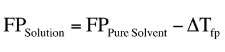# Problem: Calculate the freezing point and boiling point of each aqueous solution, assuming complete dissociation of the solute.Calculate the freezing point of the solution containing 0.111 m K2S.

###### FREE Expert Solution

To calculate the freezing point of the solution we use the formula below:The pure solvent here is just water because the solution is aqueous.

Now the ∆Tfp can be calculated with the formula below:

97% (266 ratings)###### Problem Details

Calculate the freezing point and boiling point of each aqueous solution, assuming complete dissociation of the solute.

Calculate the freezing point of the solution containing 0.111 m K2S.